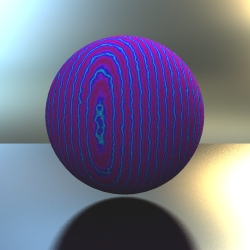Relevant to Blender v2.31

These shader blocks determine the BiDirectional Reflectivity Function (BDRF) or Illumination Model that the object is shaded with. Each different base shader type has various inputs that can receive the outputs from other shader blocks, altering the surface characteristics.

### Constant

 ``` ```

### Generic

 ``` ```

### Phong

 ``` ```

## Procedural

These Shading blocks create various procedural patterns with inline values. No inputs are needed.

### Marble

 ``` ```

• size: Size of the marble effect, lower numbers = less veins, higher numbers = more veins.

• depth: Controls the number of iterations (number of noise frequencies added to the swirl).

• hard: Controls the noise type, when set to 'off' the noise varies smoothly while setting it to 'on' will show more abrupt changes in color.

• turbulence: Controls the amount of noise turbulence.

• sharpness: Controls the sharpness of color 1 compared to color 2, the higher this value, the thinner the color band of color1. This effect is similar to the soft/sharp/sharper switches of the Blender marble texture, the difference is that it is more controlable here. The value must be at least 1 or higher.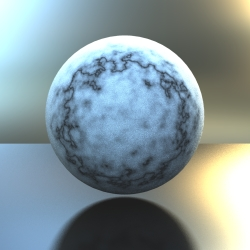### Wood

 ``` ```

• size: Size of the wood effect, lower numbers = less wood grain, higher numbers = more wood grain.

• depth: Controls the number of iterations (number of noise frequencies added to the swirl).

• hard: Controls the noise type, when set to 'off' the noise varies smoothly while setting it to 'on' will show more abrupt changes in color.

• turbulence: Controls the amount of noise turbulence.

• ringscale_x: Controls the width of the wood rings in the x axis.

• ringscale_y: Controls the width of the wood rings in the y axis.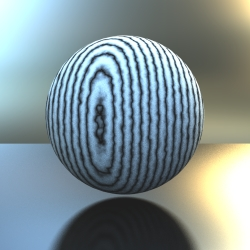### Clouds

 ``` ```

• size: Size of the cloud effect.

• depth: Controls the number of iterations (number of noise frequencies added to the swirl).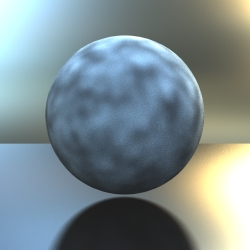These allow the modification of other shaders and the building of "chains" of simple shaders to build a complex shader.

Takes a color as input and outputs a float

 ``` ```

• input: Input (color) to convert to float.

Builds a color from a value input and a gradient. An unlimited number of modulators add nodes to the gradient. The shader interpolates the color values of the nodes at the given input value. In the example below, an input value of 0.12 would generate a colour between the first and second node, which are black and orange. So a dark orange would be the result.

 ``` ```

### Conetrace block

It can be used to get reflections or transmitted color from environment. But it could also be used to get blurry ones.

 ``` ```

• reflect: 'on' will reflect the ray, 'off' will refract the ray.

• angle: Angle of the cone (around the ray) to be sampled, 0 for a simple sharp reflection/refraction.

• samples: Number of samples to take inside the cone.

• IOR: Index of Refraction.

• color: Color to filter the incoming light.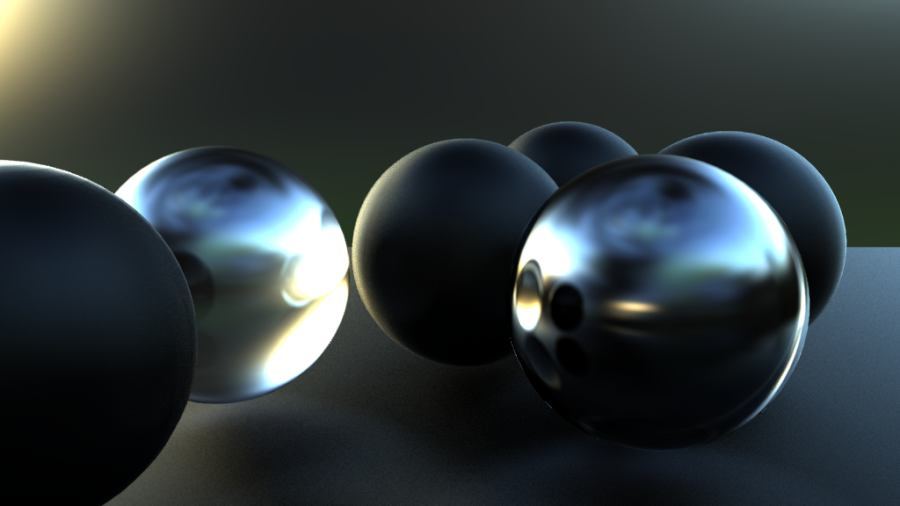Spheres with varying levels of blurry reflections and refractions and an HDRI background.

Outputs a float based on object coords.

 ``` ```

• coord: Coordinate to use, either X, Y or Z.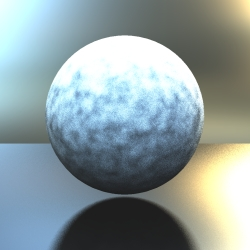Takes a float as input and outputs a color.

 ``` ```

• input: Input (float) to convert to color.

Can be used to get realistic reflections/refractions based on the angle of incidence.

 ``` ```

• reflected: The input to use as reflected color (usually the conetrace output).

• transmitted: The input to use as transmitted color (usually another conetrace output).

• IOR: Index of Refraction.

• min_refle: Minimal reflection amount.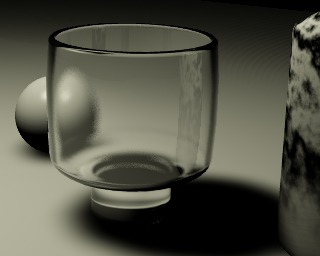Mixing fresnel and conetrace blocks.

Builds a color from either any inputs or inline values for HSV components.

 ``` ```

As in the RGB color, if the inputs are omitted, inline hue/saturation/value values are used.

Assigns a bitmap image to the object according to its UV co-ordinates (outputs Color).

 ``` ```

• filename: Path and name of bitmap to apply.

Mixes x2 inputs in different ways, depending on the mode used.

 ``` ```

• input1: First input to mix.

• input2: Second input to mix.

• mode: Possible mix modes (note, some modes output different results depending on the order of the inputs).

These are: Add, Average, Color Burn, Color Dodge, Darken, Difference, Exclusion, Freeze, Hard Light, Lighten, Multiply, Negation, Overlay, Reflect, Screen, Soft light, Stamp, Subtractive.

Multiplies (float) input values or input value and const value, outputs a float.

 ``` ```

• input1: First input to multiply.

• input2: Second input to multiply (if null, input1 is multiplied with the inline 'value' setting).

• value: Value to multiply if input2 is null.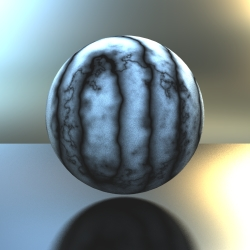Multiply shader with wood and marble as input.

Builds a color from either any inputs or inline values for RGB components.

 ``` ```

If one of the inputs is omitted, then the default color given by "color" tag is used for that input.

 ``` ```